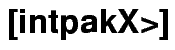Example: Interval Newton Method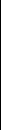The Task Let. Find enclosing intervals for all zeros of f on the interval [1.05,1.5]. The Solution Here's the Maple Worksheet solving the task with intpakX. Doing 10 iteration steps, you can prove the existence of two zeros and find two intervals which must contain every further zero of the function on the interval. Further iteration steps and a smaller parameter for the accuracy would yield more detailed results. This is to load intpakX: > restart; > libname:="/usr/maple/intpakX/lib", libname; > with(intpakX); And this is the solution: > f:=x->sin(1/(x-1));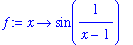> X:=[1.05,1.5];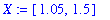> compute_all_zeros_with_plot(f,X,0.001); Enter maximal number of iteration steps: 10; `Digits = 10` `Iteration step 1` `xold= [1.05, 1.5]` `xnew1= [1.276187073, 1.5]` `xnew2= [1.05, 1.273812927]``Iteration step 2` `xold= [1.276187073, 1.5]` `xnew1= [1.276187073, 1.347259211]`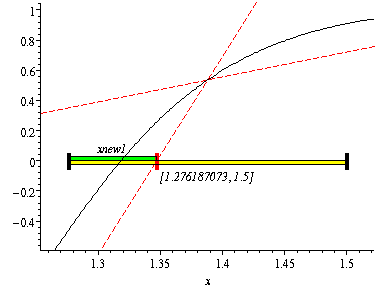`Iteration step 3` `xold= [1.276187073, 1.347259211]` `xnew1= [1.316783008, 1.320737304]`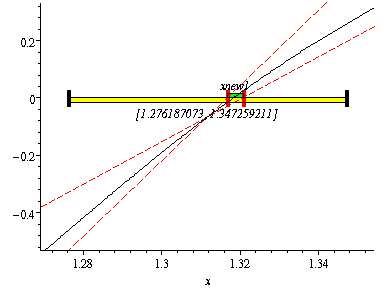`Iteration step 4` `xold= [1.316783008, 1.320737304]` `xnew1= [1.318303507, 1.318314830]`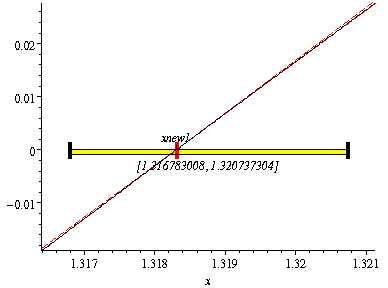`Iteration step 5` `xold= [1.05, 1.273812927]` `xnew1= [1.162172903, 1.273812927]` `xnew2= [1.05, 1.161640024]`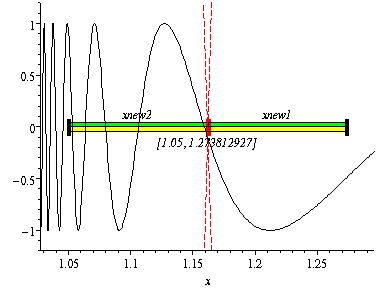`Iteration step 6` `xold= [1.05, 1.161640024]` `xnew1= [1.105883079, 1.161640024]` `xnew2= [1.05, 1.105756945]``Iteration step 7` `xold= [1.105883079, 1.161640024]` `xnew1= [1.144181348, 1.161640024]` `xnew2= [1.105883079, 1.123341755]`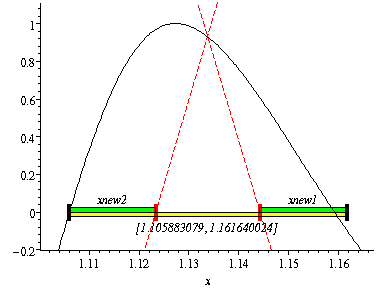`Iteration step 8` `xold= [1.144181348, 1.161640024]` `xnew1= [1.158186203, 1.161255603]`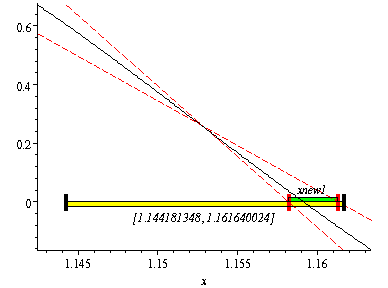`Iteration step 9` `xold= [1.158186203, 1.161255603]` `xnew1= [1.159140063, 1.159163842]``Iteration step 10` `xold= [1.105883079, 1.123341755]` `xnew1= [1.105883079, 1.107392352]`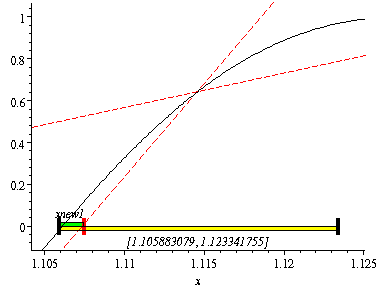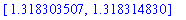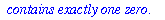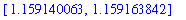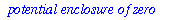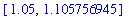`Number of enclosures of zeros: 4` `Number of iteration steps: 10` >intpakX     Wiss. Rechnen / Softwaretechnologie     FB Mathematik     Bergische Universität Wuppertal